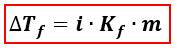# Problem: A 5 g sample of CaI2 is completely dissolved in 22 g of water, what is the freezing point of the solution? Assume complete dissociation of the salt. Kf for water is 1.86°C/m.

###### FREE Expert Solution
81% (475 ratings)
###### FREE Expert Solution

We’re being asked to find the freezing point of a solution when CaI2 is dissolved in water. When calculating the freezing point of a solution, we’re going to use the equation for Freezing Point Depression.∆Tf = change in freezing point = Tf pure solvent –Tf solution
Kf = freezing point depression constant
i = van' t Hoff factor of the solute = no. of ions
m = molality

Let’s first calculate the molality of the solution.

81% (475 ratings)###### Problem Details

A 5 g sample of CaI2 is completely dissolved in 22 g of water, what is the freezing point of the solution? Assume complete dissociation of the salt. Kf for water is 1.86°C/m.

What scientific concept do you need to know in order to solve this problem?

Our tutors have indicated that to solve this problem you will need to apply the Freezing Point Depression concept. If you need more Freezing Point Depression practice, you can also practice Freezing Point Depression practice problems.

What is the difficulty of this problem?

Our tutors rated the difficulty ofA 5 g sample of CaI2 is completely dissolved in 22 g of wate...as medium difficulty.

How long does this problem take to solve?

Our expert Chemistry tutor, Sabrina took 5 minutes and 23 seconds to solve this problem. You can follow their steps in the video explanation above.

What professor is this problem relevant for?

Based on our data, we think this problem is relevant for Professor Weng's class at YU.